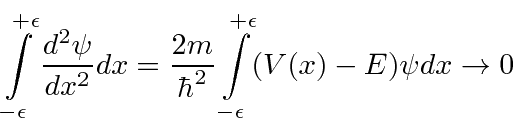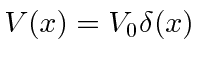### Continuity of Wavefunctions and Derivatives

We can use the Schrödinger Equation to show that the first derivative of the wave function should be continuous, unless the potential is infinite at the boundary.Integrate both sides from just below a boundary (assumed to be at) to just above.Letgo to zero and the right hand side must go to zero for finite potentials.Infinite potentials are unphysical but often handy. The delta function potential is very handy, so we will derive a special continuity equation for it. Assume. Integrating the Schrödinger Equation, we getAs before, finite terms in the right hand integral go to zero as, but now the delta function gives a fixed contribution to the integral.There is a discontinuity in the derivative of the wave function proportional to the wave function at that point (and to the strength of the delta function potential).

Jim Branson 2013-04-22